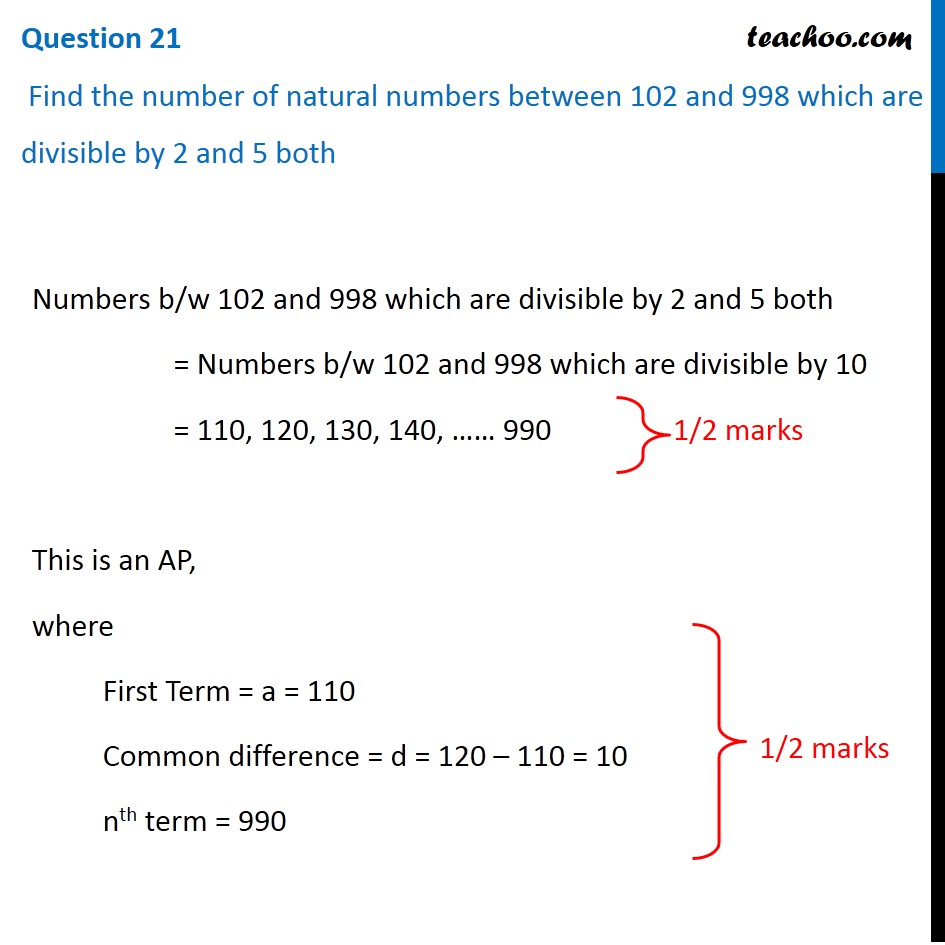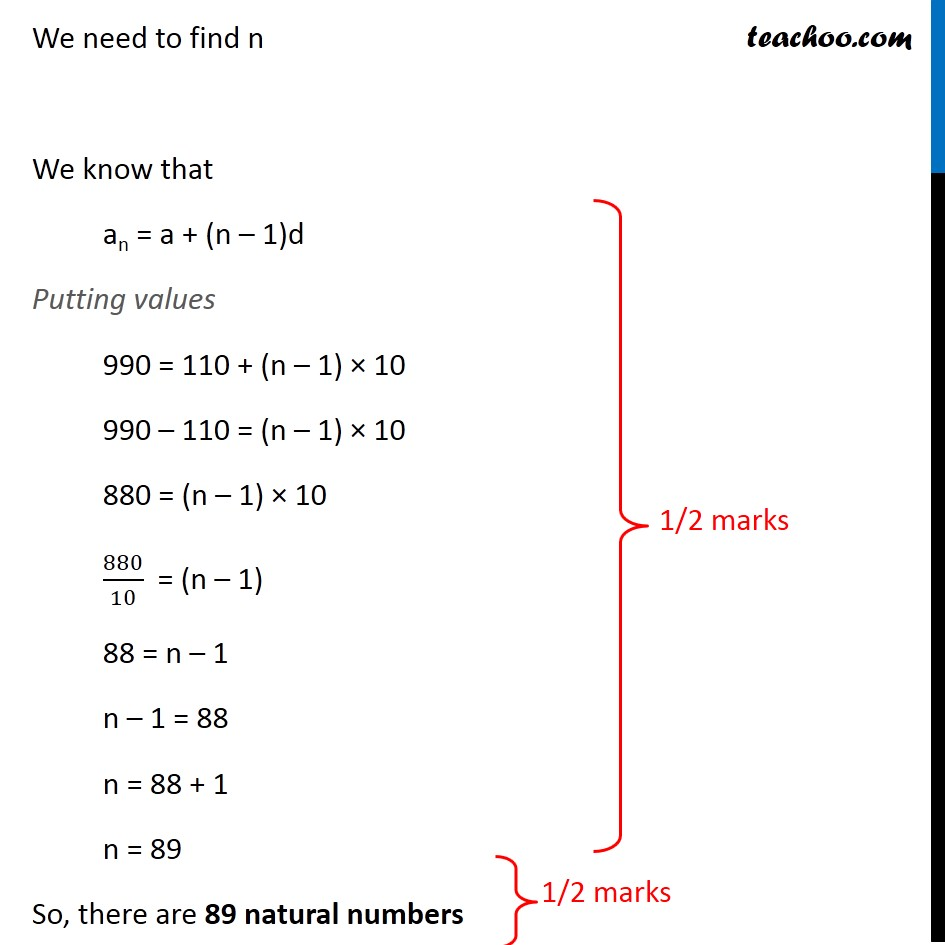CBSE Class 10 Sample Paper for 2020 Boards - Maths Standard

Class 10
Solutions of Sample Papers for Class 10 Boards

## Find the number of natural numbers between 102 and 998 which are divisible by 2 and 5 bothLearn in your speed, with individual attention - Teachoo Maths 1-on-1 Class

### Transcript

Question 21 Find the number of natural numbers between 102 and 998 which are divisible by 2 and 5 both Numbers b/w 102 and 998 which are divisible by 2 and 5 both = Numbers b/w 102 and 998 which are divisible by 10 = 110, 120, 130, 140, …… 990 This is an AP, where First Term = a = 110 Common difference = d = 120 – 110 = 10 nth term = 990 We need to find n We know that an = a + (n – 1)d Putting values 990 = 110 + (n – 1) × 10 990 – 110 = (n – 1) × 10 880 = (n – 1) × 10 880/10 = (n – 1) 88 = n – 1 n – 1 = 88 n = 88 + 1 n = 89 So, there are 89 natural numbers